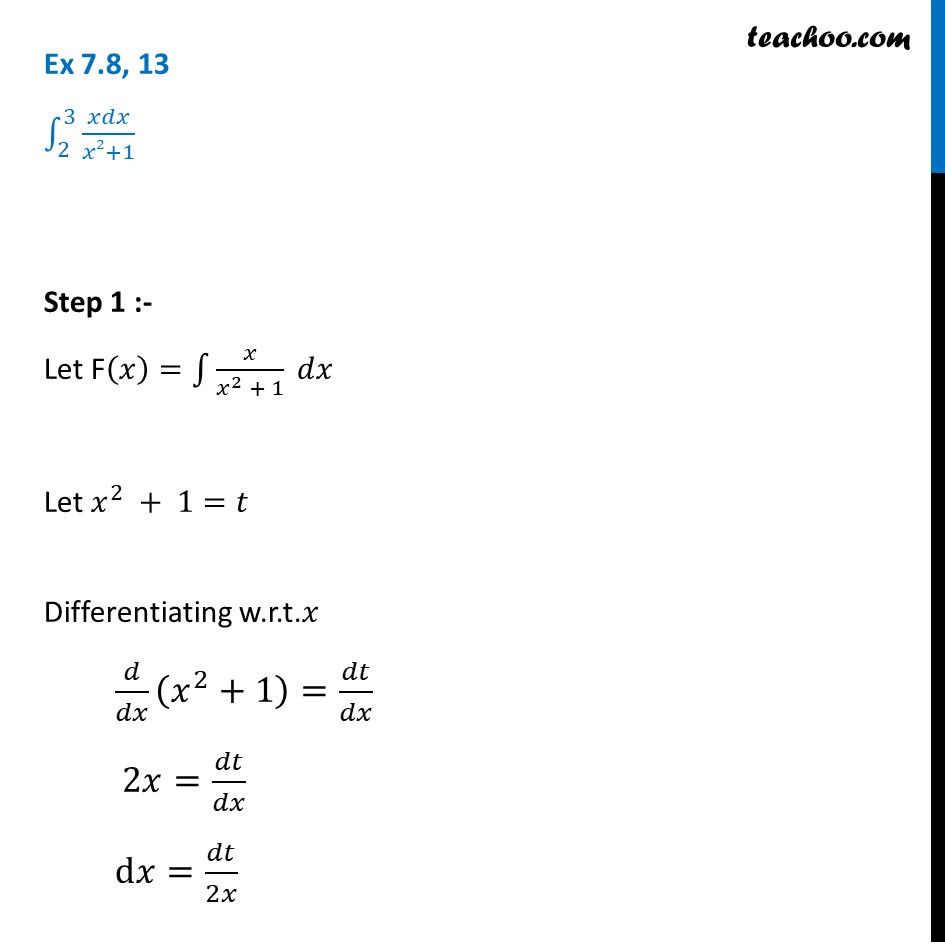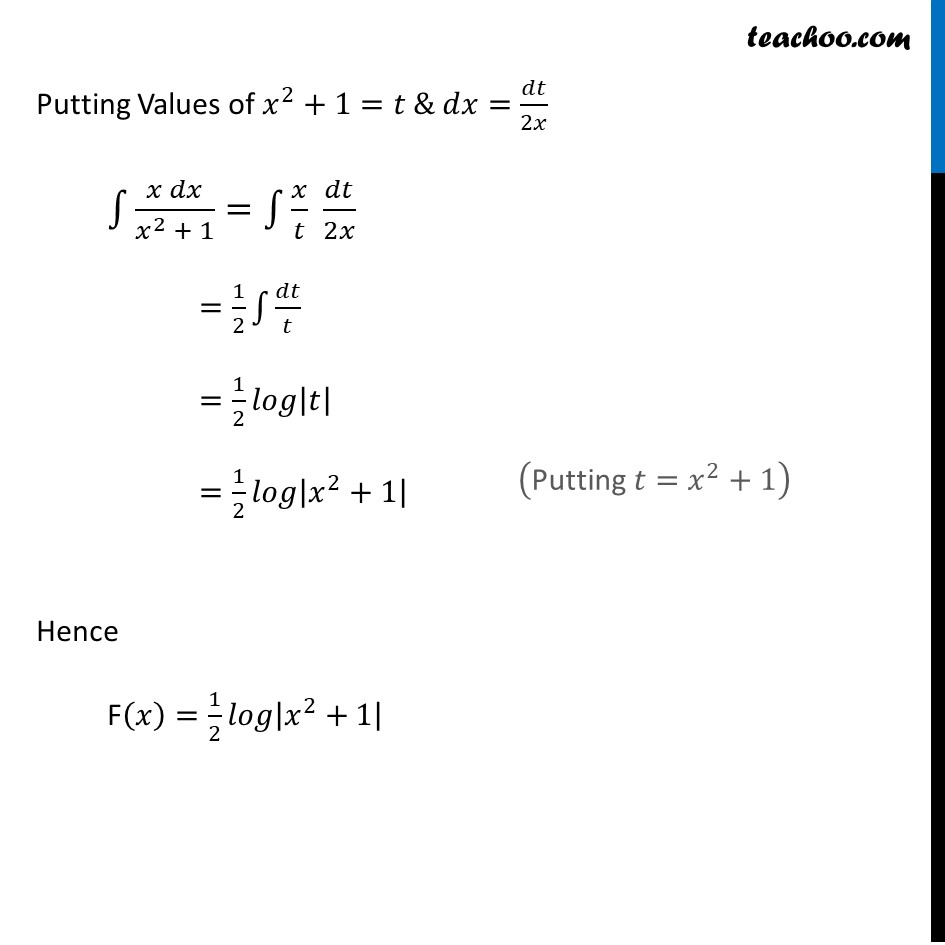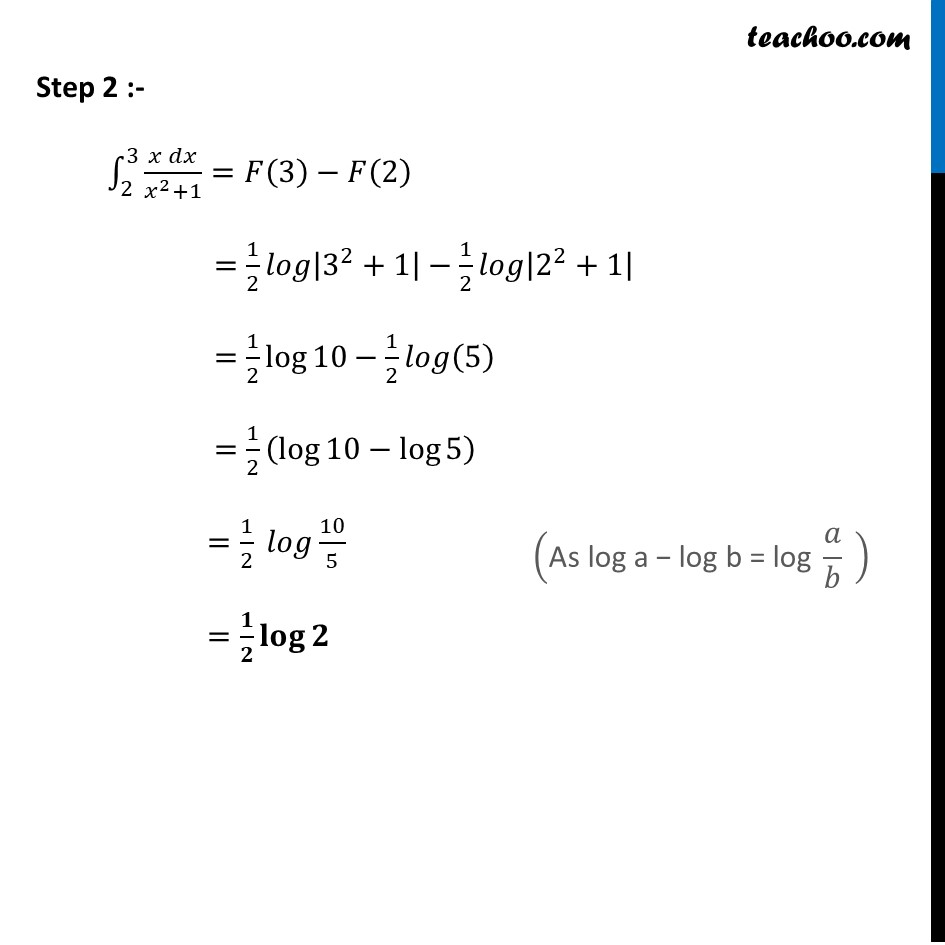Ex 7.8

Chapter 7 Class 12 Integrals
Serial order wiseLearn in your speed, with individual attention - Teachoo Maths 1-on-1 Class

### Transcript

Ex 7.8, 13 ∫_2^3▒𝑥𝑑𝑥/(𝑥2+1) Step 1 :- Let F(𝑥)=∫1▒〖𝑥/(𝑥^2 + 1) 𝑑𝑥〗 Let 𝑥^2 + 1=𝑡 Differentiating w.r.t.𝑥 𝑑/𝑑𝑥 (𝑥^2+1)=𝑑𝑡/𝑑𝑥 2𝑥=𝑑𝑡/𝑑𝑥 d𝑥=𝑑𝑡/2𝑥 Putting Values of 𝑥^2+1=𝑡 & 𝑑𝑥=𝑑𝑡/2𝑥 ∫1▒〖(𝑥 𝑑𝑥)/(𝑥^2 + 1)=∫1▒〖𝑥/𝑡 𝑑𝑡/2𝑥〗〗 =1/2 ∫1▒𝑑𝑡/𝑡 =1/2 𝑙𝑜𝑔|𝑡| =1/2 𝑙𝑜𝑔|𝑥^2+1| Hence F(𝑥)=1/2 𝑙𝑜𝑔|𝑥^2+1| Step 2 :- ∫_2^3▒(𝑥 𝑑𝑥)/(𝑥^2+1)=𝐹(3)−𝐹(2) =1/2 𝑙𝑜𝑔|3^2+1|−1/2 𝑙𝑜𝑔|2^2+1| =1/2 log⁡〖10−1/2 𝑙𝑜𝑔(5)〗 =1/2 (log⁡〖10−log⁡5 〗 ) =1/2 𝑙𝑜𝑔 10/5 =𝟏/𝟐 𝐥𝐨𝐠⁡𝟐